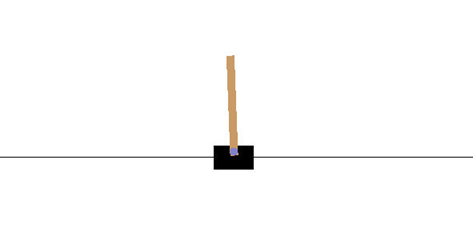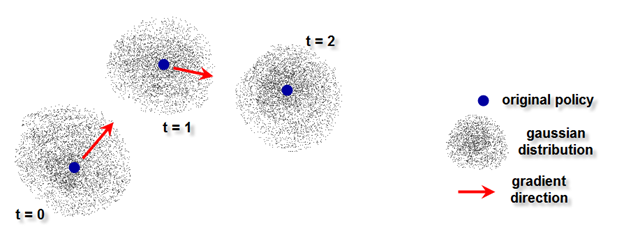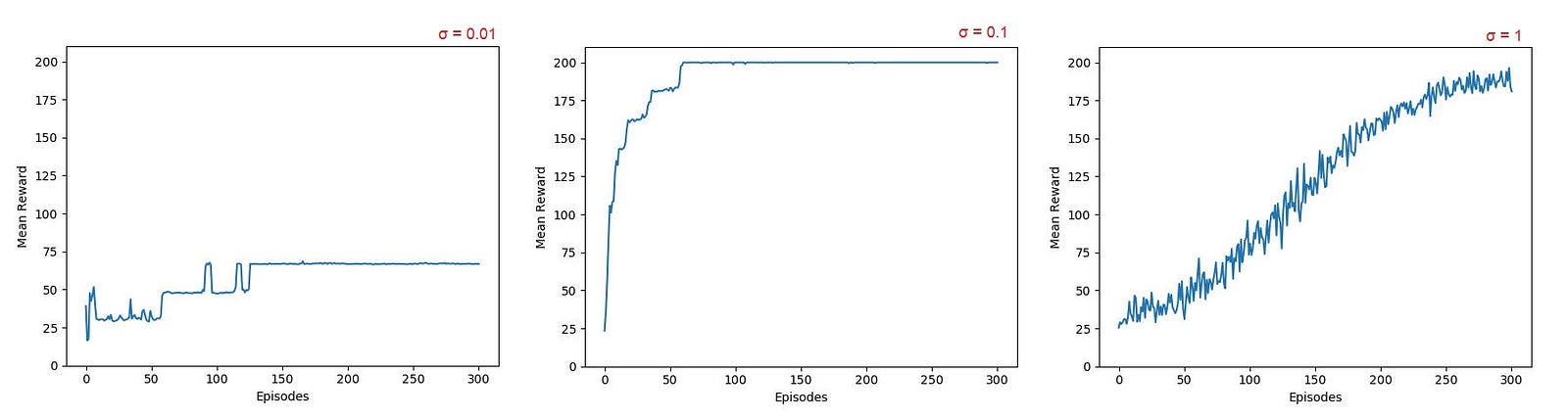# Evolution Strategies

So simple, yet so powerful

If you have been playing around with Reinforcement Learning (RL) algorithms lately you surely noticed how tricky they can be to implement correctly: calculating gradients, adding target networks, experience replay…

For sure you can always use 3rd party implementations, e.g. OpenAI Baselines, but that defeats the purpose of learning. You will never fully master an algorithm if you do not implement it yourself from scratch.

So after struggling through the details of DQN, DDPG and A3C for a while, I was incredibly excited when I discovered Evolution Strategies (ES) as a valid alternative: https://arxiv.org/abs/1703.03864

I will attempt to explain ES in simple terms here and show the implementation of this technique to solve the standard CartPole environment on Gym.CartPole environment on Gym

ES is very similar to other policy search algorithms:

1. you have a neural network that represents the policy, i.e. a function that decides what action to take given the current state

2. you need to improve this policy so that the total reward is maximized

The difference is in how you improve the policy. While other RL methods explore the action world by trying different random actions and essentially reinforce the good ones, ES works directly on the parameters of the network and randomly jitters them around hoping to find good outcomes.

ES is often dubbed a “black-box” optimization: we do not need to know anything about how the policy works. It is a box with an input (state) and output (actions) and we move around the knobs (weights) until we find a better solution, hopefully the optimal solution.

Given an initial policy (either random or pre-learned through supervised learning) we can always generate a population of similar policies around it by applying random perturbations to its weights. We then evaluate all these new policies and estimate the gradient, that is, we check in what direction things look more promising. Finally, we update the initial weights to move exactly in that direction and start again from there. We loop until we are happy with the outcome.Evolution follows the best path

You see, this algorithm is so incredibly easy, just three actions in a loop, yet it is so powerful.

Some advantages are:

1. No back-propagation is needed, which makes the implementation super easy and the execution super fast.

2. No differentiable policy is needed; you can use any function approximation you want, even binary ones, because no complicated gradients are to be calculated on the network.

3. No massive memory required to store all episodes for future updates, e.g. experience replay.

4. No value function is needed, only define one network for the policy and that’s all.

5. Better exploration behavior than other policy search techniques. Apparently, by tweaking the weights directly you can generate “more random behavior” than by tweaking the actions.

6. Given its simplicity and lack of massive internal data exchange it is extremely scalable, which means it can be easily parallelized and executed very fast on a large number of CPUs.

7. Invariant to the sampling time of observations, that is, it does not matter how often the actions are performed and rewards calculated.

There are disadvantages as well, for example that more data is usually needed, and especially that the environment’s rewards need to change quickly enough with different network weights, otherwise the policy stays stuck somewhere and will never learn anything (although you can always increase the variance of the Gaussian distribution, as we will see later).

Now, let’s see how to put ES into code:

• We need a network for our policy with a forward propagation function. We chose a small network for our simple example (1 hidden layer with 20 nodes), but you can quickly change the capacity of the network according to the complexity of the policy.
`import numpy as npimport gym`
`#neural network to store state to action policy#add hidden layers or nodes according to needsIL = 4 #input layer nodesHL = 20 #hidden layer nodesOL = 2 #output layer nodesw1 = np.random.randn(HL,IL) / np.sqrt(IL)w2 = np.random.randn(OL,HL) / np.sqrt(HL)NumWeights1 = len(w1.flatten())NumWeights2 = len(w2.flatten())`
`#forward propagationdef predict(s,w1,w2):    h = np.dot(w1,s) #input to hidden layer    h[h<0]=0 #relu    out = np.dot(w2,h) #hidden layer to output    out = 1.0 / (1.0 + np.exp(-out)) #sigmoid     return out`
• Next, we load the environment and define some hyper-parameters. The number of policies decides how many random variations around the original policy are generated and evaluated. If set too high it will take too long to run, if set too low it will not explore the environment enough. Sigma determines how far away from the original policy we will explore (see below for explanation).
`#load environmentenv = gym.make('CartPole-v0')`
`#parametersNumEpisodes = 50NumPolicies = 10sigma = 0.1learning_rate = 0.001`
`Reward = np.zeros(NumPolicies)`
• Now we start the main loop, and generate a new group of policies randomly distributed around the original one:
`#start learningfor episode in range(NumEpisodes):`
`    #generate random variations around original policy    eps = np.random.randn(NumPolicies,NumWeights1+NumWeights2)`
`    #evaluate each policy over one episode    for policy in range(NumPolicies):`
`        w1_try = w1 + sigma * eps[policy,:NumWeights1].reshape(w1.shape)        w2_try = w2 + sigma * eps[policy,NumWeights1:].reshape(w2.shape)`
• Each of those new policies need to be evaluated, i.e. we run the agent in the environment and collect rewards:
`    #initial state    observation = env.reset() #observe initial state`
`    Reward[policy] = 0`
`    while True:`
`        Action = predict(observation,w1_try,w2_try)        Action = np.argmax(Action)`
`        #execute action        observation_new, reward, done, _ = env.step(Action)         #collect reward        Reward[policy] += reward`
`        #update state        observation = observation_new`
`        #end episode        if done:            break`
`#calculate incremental rewardsF = (Reward - np.mean(Reward))`
• Finally, we update the original policy moving towards the direction that provided best results. It is a sort of gradient ascent, except that the gradient is not exactly calculated, only estimated as an average of values, and the weights are not updated through back-propagation, only incremented.
`#update weights of original policy according to rewards of all variationsweights_update = learning_rate/(NumPolicies*sigma) * np.dot(eps.T, F)`
`w1 += weights_update[:NumWeights1].reshape(w1.shape)w2 += weights_update[NumWeights1:].reshape(w2.shape)`

Note 1: What does this technique have to do with evolution? Well, you can think of your policy as a living being and the jittering of its parameters as random mutations in its DNA over time. Each mutation generates a new being, which will be either “better” or “worse” than the original, where being better or worse means receiving from the environment more or less reward during a lifetime. The algorithm highlights the mutations that accumulated larger rewards and moves the next generation of beings in that direction. It’s the law of nature, the stronger will survive, the weaker will perish.

Note 2: Is Sigma important? Yes, all hyper-parameters play a role in determining how effecting the algorithm learns. We generate new individuals randomly distributed around the original one. Sigma decides how strong the mutations are, in other words how much we explore around the parameters world. With very low Sigma the behavior of the new policies is essentially the same as the previous one, which means not much can be learned at all. With very large Sigma the new policies are spread out too far apart from each other and it can be difficult to decide where to move on next, resulting in slow improvements as well. The following graphs show the learning curves averaged over 10 different runs for Sigma = 0.01 (almost no learning); 0.1 (very quick learning); 1 (very slow learning).Too little vs. too much exploration

Note 3: while it is perfectly possible and correct to set the input of the policy network equal to the raw observed state, it is always a good idea to normalize the input values and accelerate the learning process:

`                #normalize inputs                observation /= 2.5                observation /= 2.5                observation /= 0.2                observation /= 2.5`